International
Tables for
Crystallography
Volume B
Reciprocal space
Edited by U. Shmueli

International Tables for Crystallography (2006). Vol. B. ch. 1.2, p. 15   | 1 | 2 |

## Section 1.2.7.2. Reciprocal-space description of aspherical atoms

P. Coppensa*

aDepartment of Chemistry, Natural Sciences & Mathematics Complex, State University of New York at Buffalo, Buffalo, New York 14260-3000, USA
Correspondence e-mail: coppens@acsu.buffalo.edu

#### 1.2.7.2. Reciprocal-space description of aspherical atoms

| top | pdf |

The aspherical-atom form factor is obtained by substitution of (1.2.7.6)in expression (1.2.4.3a):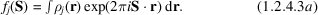In order to evaluate the integral, the scattering operator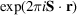must be written as an expansion of products of spherical harmonic functions. In terms of the complex spherical harmonic functions, the appropriate expression is (Weiss & Freeman, 1959; Cohen-Tannoudji et al., 1977)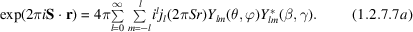The Fourier transform of the product of a complex spherical harmonic function with normalization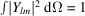and an arbitrary radial function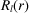follows from the orthonormality properties of the spherical harmonic functions, and is given by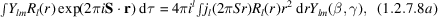where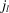is the lth-order spherical Bessel function (Arfken, 1970), and θ and ϕ, β and γ are the angular coordinates of r and S, respectively.

For the Fourier transform of the real spherical harmonic functions, the scattering operator is expressed in terms of the real spherical harmonics: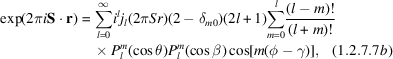which leads to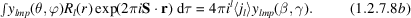Since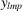occurs on both sides, the expression is independent of the normalization selected. Therefore, for the Fourier transform of the density functions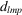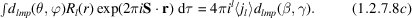In (1.2.7.8b)and (1.2.7.8c),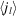, the Fourier–Bessel transform, is the radial integral defined as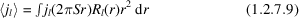of which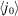in expression (1.2.4.3)is a special case. The functionsfor Hartree–Fock valence shells of the atoms are tabulated in scattering-factor tables (IT IV, 1974). Expressions for the evaluation ofusing the radial function (1.2.7.5ac) have been given by Stewart (1980)and in closed form for (1.2.7.5a)by Avery & Watson (1977)and Su & Coppens (1990). The closed-form expressions are listed in Table 1.2.7.4.

 Table 1.2.7.4| top | pdf | Closed-form expressions for Fourier transform of Slater-type functions (Avery & Watson, 1977; Su & Coppens, 1990)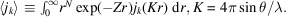N
k 1 2 3 4 5 6 7 8
0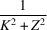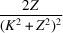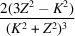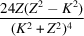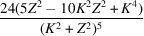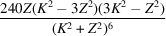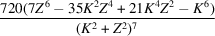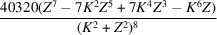1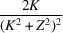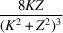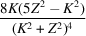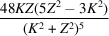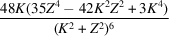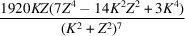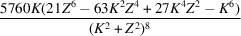2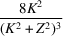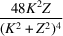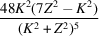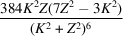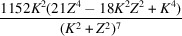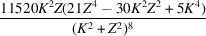3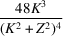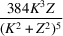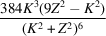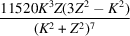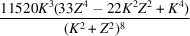4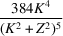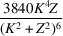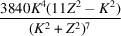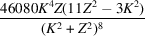5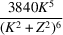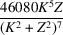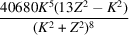6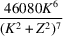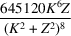7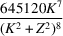Expressions (1.2.7.8)show that the Fourier transform of a direct-space spherical harmonic function is a reciprocal-space spherical harmonic function with the same l, m, or, in other words, the spherical harmonic functions are Fourier-transform invariant.

The scattering factors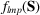of the aspherical density functions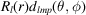in the multipole expansion (1.2.7.6)are thus given by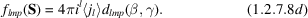The reciprocal-space spherical harmonic functions in this expression are identical to the functions given in Table 1.2.7.1, except for the replacement of the direction cosines x, y and z by the direction cosines of the scattering vector S.

### ReferencesInternational Tables for X-ray Crystallography (1974). Vol. IV. Birmingham: Kynoch Press. (Present distributor Kluwer Academic Publishers, Dordrecht.)Google ScholarArfken, G. (1970). Mathematical models for physicists, 2nd ed. New York, London: Academic Press.Google ScholarAvery, J. & Watson, K. J. (1977). Generalized X-ray scattering factors. Simple closed-form expressions for the one-centre case with Slater-type orbitals. Acta Cryst. A33, 679–680.Google ScholarCohen-Tannoudji, C., Diu, B. & Laloe, F. (1977). Quantum mechanics. New York: John Wiley and Paris: Hermann.Google ScholarStewart, R. F. (1980). Electron and magnetization densities in molecules and solids, edited by P. J. Becker, pp. 439–442. New York: Plenum.Google ScholarSu, Z. & Coppens, P. (1990). Closed-form expressions for Fourier– Bessel transforms of Slater-type functions. J. Appl. Cryst. 23, 71–73.Google ScholarWeiss, R. J. & Freeman, A. J. (1959). X-ray and neutron scattering for electrons in a crystalline field and the determination of outer electron configurations in iron and nickel. J. Phys. Chem. Solids, 10, 147–161.Google Scholar